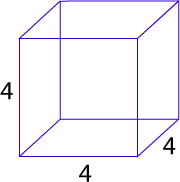# Volume Of Cube Java Program – 2 Ways | Programs

Java program to calculate the volume of a cube – In this distinct piece, we will talk more about the different ways to calculate the volume of a cube in Java Programming.

Fitting examples and sample programs have been added for the sake of greater comprehension for interested people. The compiler has also been added with which you can execute yourself.

The means with which the volume of a cube is calculated in this piece in Java programming are as follows:

• Using Scanner Class
• Using Command Line Arguments
• Using Static Function

As we all know, a cube is a three-dimensional figure which is used every day in our daily life. A cube is just an extrapolation of a square by giving it a height of the same length.

All the sides of a cube are equal and the opposite sides are parallel. All the angles in a cube are equal to 90 degrees.

This is what a cube looks like:As you can see, this is a cube with a side of 4 units. The volume of a cube can be calculated with this formula:

Volume = Side*Side*Side

Hence, the volume of this cube is as follows:

Volume = 4*4*4 = 64 cubic units.

Thus, the multitude of ways to calculate the volume of a cube in Java Programming is as follows:

Output:

Output:

Output:
x

## Plus Star Pattern Java Program | Patterns

Java program to print plus star pattern program – We have written the below print/draw ...